# Triangles - Olympiad Level MCQ, Class 10 Mathematics

## 25 Questions MCQ Test Olympiad Preparation for Class 10 | Triangles - Olympiad Level MCQ, Class 10 Mathematics

Description
Attempt Triangles - Olympiad Level MCQ, Class 10 Mathematics | 25 questions in 25 minutes | Mock test for Class 10 preparation | Free important questions MCQ to study Olympiad Preparation for Class 10 for Class 10 Exam | Download free PDF with solutions
QUESTION: 1

Solution:
QUESTION: 2

Solution:
QUESTION: 3

### The point of intersection of the angle bisectors of a triangle is :

Solution:
QUESTION: 4

A triangle PQR is formed by joining the mid-points of the sides of a triangle ABC. 'O' is the circumcentre of

ΔABC, then for ΔPQR, the point 'O' is :

Solution:
QUESTION: 5

If AD, BE, CF are the altitudes of ΔABC whose orthocentre is H, then C is the orthocentre of :

Solution:
QUESTION: 6

In an equilateral ΔABC, if a, b and c denote the lengths of perpendiculars from A, B and C respectively on the opposite sides, then:

Solution:
QUESTION: 7

Any two of the four triangles formed by joining the midpoints of the sides of a given triangle are:

Solution:
QUESTION: 8

The internal bisectors of ∠B and ∠C of ΔABC meet at O. If ∠A = 80° then ∠BOC is :

Solution:
QUESTION: 9

The point in the plane of a triangle which is at equal perpendicular distance from the sides of the triangle is :

Solution:
QUESTION: 10

Incentre of a triangle lies in the interior of :

Solution:
QUESTION: 11

In a triangle PQR, PQ = 20 cm and PR = 6 cm, the side QR is :

Solution:
QUESTION: 12

If ABC is a right angled triangle at B and M, N are the mid-points of AB and BC, then 4 (AN2 + CM2) is equal to–

Solution:
QUESTION: 13

ABC is a right angle triangle at A and AD is perpendicular to the hypotenuse. Then BD/CD is equal to :

Solution:
QUESTION: 14

Let ABC be an equilateral triangle. Let BE . CA meeting CA at E, then (AB2 + BC2 + CA2) is equal to :

Solution:
QUESTION: 15

If D, E and F are respectively the mid-points of sides of BC, CA and AB of a ΔABC. If EF = 3 cm, FD =

4 cm, and AB = 10 cm, then DE, BC and CA respectively will be equal to :

Solution:
QUESTION: 16

In the right angle triangle ∠C = 90°. AE and BD are two medians of a triangle ABC meeting at F. The ratio

of the area of ΔABF and the quadrilateral FDCE is :

Solution:
QUESTION: 17

The bisector of the exterior ∠A of ΔABC intersects the side BC produced to D. Here CF is parallel to AD.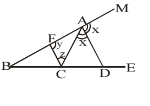Solution:
QUESTION: 18

The diagonal BD of a quadrilateral ABCD bisects ∠B and ∠D, then: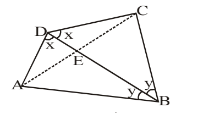Solution:
QUESTION: 19

Two right triangles ABC and DBC are drawn on the same hypotenuse BC on the same side of BC. If AC and

DB intersects at P, then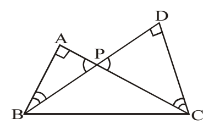Solution:
QUESTION: 20

In figure, ABC is a right triangle, right angled at B. AD and CE are the two medians drawn from A and C

respectively. If AC = 5 cm and AD =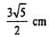, find the length of CE: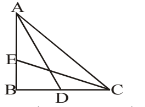Solution:

Given: A triangle ABC right angled at B. AD and CE and medians drawn from vertices A and C respectively.
To find: CE = ?
In ∆ABD, AD2 = AB2 + BD2
[Using Pythagoras theorem]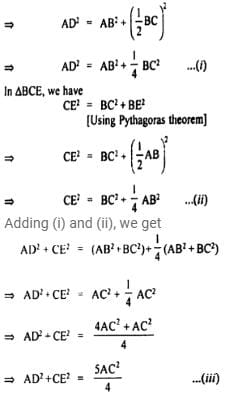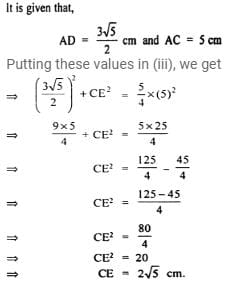QUESTION: 21

In a ΔABC, AB = 10 cm, BC = 12 cm and AC = 14 cm. Find the length of median AD. If G is the centroid,

find length of GA :

Solution:
QUESTION: 22

The three sides of a triangles are given. Which one of the following is not a right triangle?

Solution:
QUESTION: 23

In the figure AD is the external bisector of ∠EAC, intersects BC produced to D. If AB = 12 cm, AC = 8 cm

and BC = 4 cm, find CD.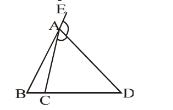Solution:
QUESTION: 24

In ΔABC, AB+ AC2 = 2500 cm2 and median AD = 25 cm, find BC.

Solution:
QUESTION: 25

In the given figure, AB = BC and ∠BAC = 15°, AB = 10 cm. Find the area of ΔABC.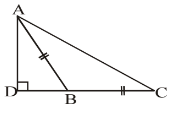Solution:Use Code STAYHOME200 and get INR 200 additional OFF Use Coupon Code